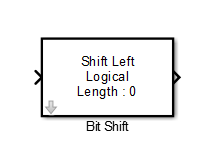Bit Shift

Logical or arithmetic shift of input signal

Library

HDL Coder / Logic and Bit Operations

•Description

The Bit Shift block performs a logical or arithmetic shift on the input signal.

This block is different from the Shift Arithmetic block in terms of simulation and HDL code generation behavior. The Bit Concat block can perform logical shifting of a signed number without having to perform a reinterpretcast operation. This block uses a MATLAB Function block based implementation and might be slower in operation.

The Shift Arithmetic block shifts the bits or binary point of the input number. This block has additional block options for HDL code generation in comparison to the Bit Concat block. When you want to perform a variable shift operation, use the Shift Arithmetic block instead of the Bit Concat block. If you have a signed number as input, the block performs a sign extension of the number. The Shift Arithmetic block requires using additional Data Type Conversion blocks that have the Stored Integer (SI) option selected.

Parameters

Shift Mode

Default: Shift Left Logical

Specifies the type and direction of shift:

• Shift Left Logical (default)

• Shift Right Logical

• Shift Right Arithmetic

Shift Length

Specifies the number of bits to be shifted. Specify a value greater than or equal to zero. The default is 0.

Ports

The block has the following ports:

Input
• Supported data types: Fixed-point, integer (signed or unsigned), Boolean

• Minimum bit width: 2

• Maximum bit width: 128

Output

Has the same data type and bit width as the input signal.